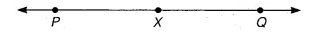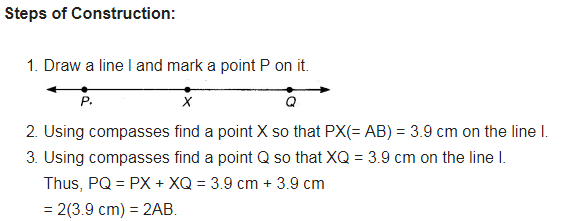# Given AB of length 3.9 cm, construct PQ such that the length of PQ

Given$\overline { AB }$ of length 3.9 cm, construct$\overline { PQ }$ such that the length of$\overline { PQ }$ is twice that of$\overline { AB }$. Verify by measurement.